Courses

# RD Sharma Solutions -Ex-15.1 & 15.2, Pair Of Lines And Transversal, Class 6, Maths Class 6 Notes | EduRev

## Class 6 : RD Sharma Solutions -Ex-15.1 & 15.2, Pair Of Lines And Transversal, Class 6, Maths Class 6 Notes | EduRev

The document RD Sharma Solutions -Ex-15.1 & 15.2, Pair Of Lines And Transversal, Class 6, Maths Class 6 Notes | EduRev is a part of the Class 6 Course RD Sharma Solutions for Class 6 Mathematics.
All you need of Class 6 at this link: Class 6

Question 1. Identify parallel line segments:

i)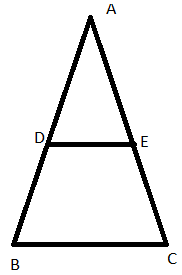ii)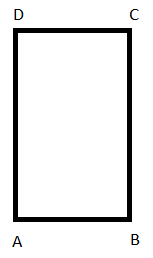iii)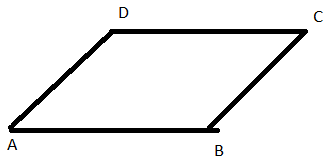iv)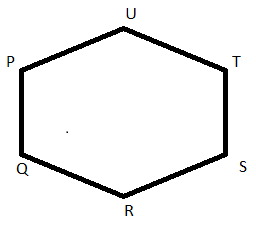v)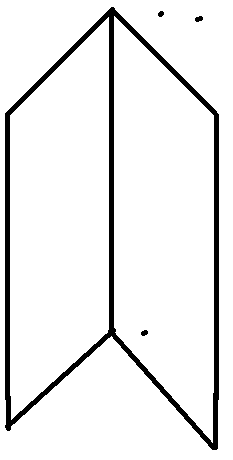vi)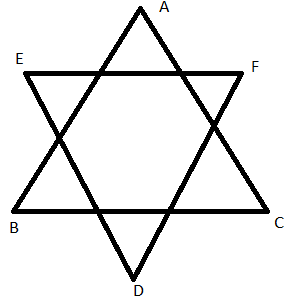1. BC ║ DE
2. AB ║  DC , AD ║  BC
3. AB ║ DC , AD ║ BC
4. PQ ║ TS , UT ║QR , UP ║ SR
5. AB ║ DC ║  EF , AD ║ BC and DE║  CF
6. BC ║  EF , AB ║  DF and AC ║  DE

Question 2. Name the pairs of all possible parallel edges of the pencil box whose figure is shown in the figure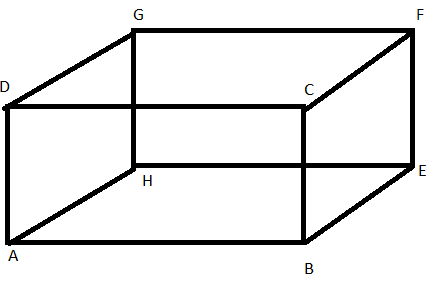AH║DG║CF║BE

AB║DC?GF║HE

Question 3. In the figure, do the segments AB and CD intersect ? are they parallel? Give reasons.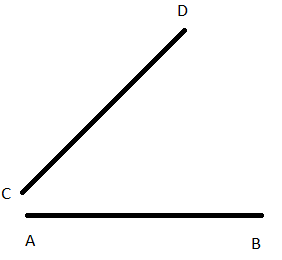In the given position , segments AB and CD do not intersect , but hey can if extended to a point . No, they are not parallel, as the dstance between them is not constant.

Question 4. State which of the following are true or false:

i) If two lines in the same plane do not intersect , then they must be parallel
Ans. True

ii) Distance between two parallel lines is not same everywhere
Ans. False

iii) If m perpendicular l and n perpendicular l and m ? n , then m parallel to n
Ans. True

iv) Two non Ans. intersecting co Ans.planar rays are parallel
Ans. False

iv) If Ray AB parallel to m , then line segment AB parallel to m
Ans. True

v) If Ray AB parallel to m , then line segment AB parallel to m
Ans. True

vi) No two parallel segments intersect each other
Ans. True

vii) Every pair of lines is a pair of co-planar lines
Ans. False

viii) Two lines perpendicular to the same line are parallel
Ans. True

ix) A line perpendicular to one of two parallel lines is perpendicular to each other
Ans. True

Exercise 15.2

Question 1. i) Alternate corresponding angles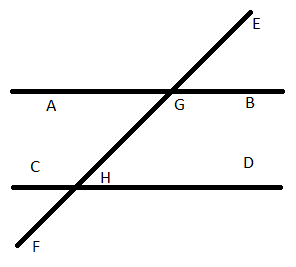Alternate interior angles are:

Angle BGH and angle CHG

Angle AGH and angle CHF

Alternate exterior angles:

Angle AGE and angle DHF

Angle EGB and angle CHF

Corresponding angles are:

Angle EGB and angle GHD

Angle EGA and angle GHC

Angle BGH and angle DHF

Angle AGF and angle CHF

ii) Angles alternate to ∠d and ∠g and angles corresponding to angles ∠f and ∠h in the figure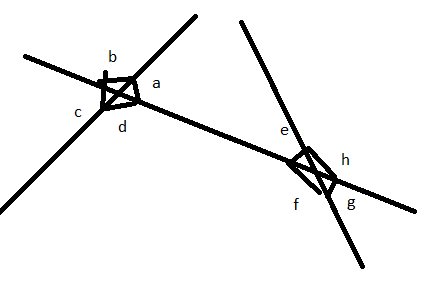The alternate angle to ∠d is ∠e and alternate angles to ∠g is ∠b

The corresponding angles to ∠f is ∠c and ∠h is ∠a

iii) Angles alternative to ∠PQR , angle corresponding to ∠RQF and angle alternative to ∠PQE in the figure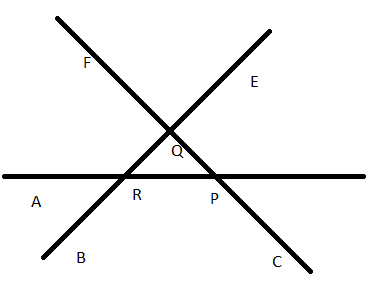In the given figure. ‘I’ is a transversal to ‘m’ and ‘n’

So, the alternate angle of ∠PQR is ∠QRA

The corresponding angle ∠RQF and ∠BRA

The alternate angle of ∠PQE is ∠BRA

iv) Interior angles on the same side of the transversal line ‘n’ are ∠d and ∠f , ∠a and ∠e

Exterior angles on the same side of the transversal line ‘n’ are ∠c and ∠g , ∠b and ∠h

Question 2. Match column A and column B .

i) Vertically opposite angles - c - ∠PAB and ∠XAQ

ii) Alternate angles - a - ∠PAB and ∠ABS

iii) Corresponding angles – b - ∠PAB and ∠RBY

Offer running on EduRev: Apply code STAYHOME200 to get INR 200 off on our premium plan EduRev Infinity!

103 docs

,

,

,

,

,

,

,

,

,

,

,

,

,

,

,

,

,

,

,

,

,

,

,

,

,

,

,

,

,

,

;